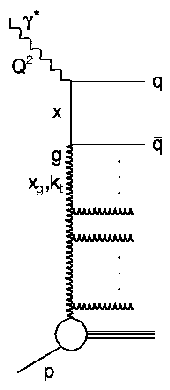back to overview of this paper

# Inclusive Dijet Production at Low Bjorken-x      in Deep Inelastic ScatteringUnderstanding the parton densities of the proton and how they evolve with kinematic variables at low values of the Bjorken variable x has been a major theme of HERA physics and QCD in recent times. The evolution of the parton densities with the factorization scale, µ², is generally described by the DGLAP equations. To leading logarithmic accuracy, this is equivalent to the exchange of a parton cascade (see figure on the right), with the exchanged partons strongly ordered in virtuality up to Q², the modulus of the squared four momentum of the photon exchanged in the ep scattering. The DGLAP approximation is, however, expected to break down at low x, as it only resums leading logarithms in Q² and neglects contributions from log (1/x) terms, which are present in the full perturbative expansion.

At very low values of x, it is believed that the theoretically most appropriate description is given by the BFKL evolution equations. These resum large logarithms of 1/x up to all orders and impose no restriction on the ordering of the transverse momenta within the parton cascade. To account for the possibly significant transverse momentum of the incoming parton to the hard scatterng, off-shell matrix elements have to be used together with an unintegrated gluon distribution function, which depends on the parton pT as well as the usual x and Q2 variables. A promising approach to parton evolution at both small and large values of x is given by the CCFM evolution equation, which, by means of angular-ordered parton emission, is equivalent to the BFKL ansatz for x -> 0, whilst reproducing the DGLAP equations at large x.

Dijet data may be used to gain insight into the dynamics of the parton cascade typically exchanged in low-x lepton-proton interactions. In the low x region, the gluon density becomes very large and photon-gluon fusion is the dominant underlying process for dijet production. Such measurements are thus sensitive to the dynamics of the parton cascade and the unintegrated gluon distribution.

The measurement presented in this paper is based on data collected with the H1 detector at HERA during the years 1996 and 1997 and covers the kinematic region of 5 < Q² < 100 GeV² and 10-4 < x < 10-2. At least two jets with transverse energies of E_T > 7 GeV and E_T > 5 GeV, well measured with the H1 Liquid Argon Calorimeter, are required. Dijet cross sections are measured as a function of x, Q², E_T and the pseudorapidity distance between the jets. The results are compared with QCD dijet calculations at next-to-leading-order. Within the uncertainties, good agreement between the data and the theoretical predictions is observed, even in regions where effects due to small-x dynamics should be most prominent.

More insight into small-x dynamics can be gained from the dijet data by studying the behaviour of events with a small azimuthal separation, Delta_phi, between the two highest transverse momentum jets. Partons entering the hard scattering process with negligible transverse momentum, as assumed in the DGLAP formalism, lead at leading order of QCD to a back-to-back configuration of the two outgoing jets with Delta_phi=180°. Azimuthal jet separations different from 180° occur due to higher order QCD effects. However, in models which predict a significant proportion of partons entering the hard process with large transverse momentum, the number of events with small Delta_phi also increases. In order to search for such effects, we present a measurement of the ratioof the number of events N_dijet with an azimuthal jet separation of Delta_phi < 120° relative to all dijet events. The result of the measurement is shown in the following figure as a function of x for different regions in Q².The fraction of jets with an azimuthal separation Delta_phi < 120° is of the order of of 5% and increases with decreasing x. This rise is most prominent in the lowest Q² interval, where the smallest values of x are reached. The NLO dijet QCD calculations predict S values of only ~1% and show no rise towards small x. The data are also compared with an NLO calculation for 3-jet production, corresponding to an extra order in the strong coupling constant alpha_s for the perturbative calculation. The 3-jet calculation is closer to the data and shows the correct qualitative features. However, it is still insufficient to describe the magnitude of S at the lowest x and Q2 values, suggesting that further improvements to the theory are required.

The data are also compared with the predictions of the CASCADE Monte Carlo program (not shown here), which includes CCFM evolution and unintegrated parton densities. The predictions are found to be highly sensitive to the choice of unintegrated gluon density, with different parameterisations that descibe the proton structure function F2 yielding rather different predictions for S. One of the unintegrated gluon parameterisations (J2003) describes the S data rather well. Hence, the S observable has a strong potential to constrain the unintegrated parton densities.